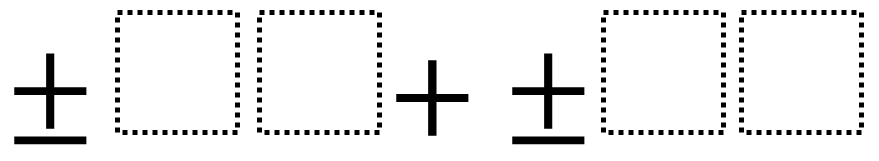Home > Grade 7 > Adding Two-Digit Numbers (Middle School)

# Adding Two-Digit Numbers (Middle School)

Directions: Make the smallest (or largest) sum by filling in the boxes using the whole numbers 1-9 no more than one time each### Hint

What does the number on the left represent?  What does the number on the right represent?

97 + 86 is one answer for the largest sum.  If only positive integers are used, then 13 + 24 is the smallest sum. If positive or negative integers are used, then -97 + -86 is the smallest sum.

Note: This problem’s difficulty can be adjusted by altering the number of digits (boxes), picking smallest or largest, or by picking either a positive, negative, or both.

Source: Robert Kaplinsky

## Converting a Fraction to a Decimal

Directions: Using the digits 1 to 9, at most one time each, place a digit …

1.Hi Robert,
I am going to suggest a better question – what is the 3rd Largest/smallest number you can make. I believe this is much more cognitively demanding and actually allows for more discussion/debate over place value. Let me know what you think.
Damian

•I love your suggestion. Gosh, that would sure make it even more challenging. Please submit it!

2.Am I reading the question wrong? Can’t I choose whether to use a positive or negative for each number? If so, what about -19 + 23 for the smallest positive sum?

•Thanks Robyn. Clearly the language being used could be more precise. What I meant by “smallest positive sum” or “smallest negative sum” was the smallest sum using only positive numbers or the smallest sum if positive or negative numbers could be used. I’ll clean up the language.